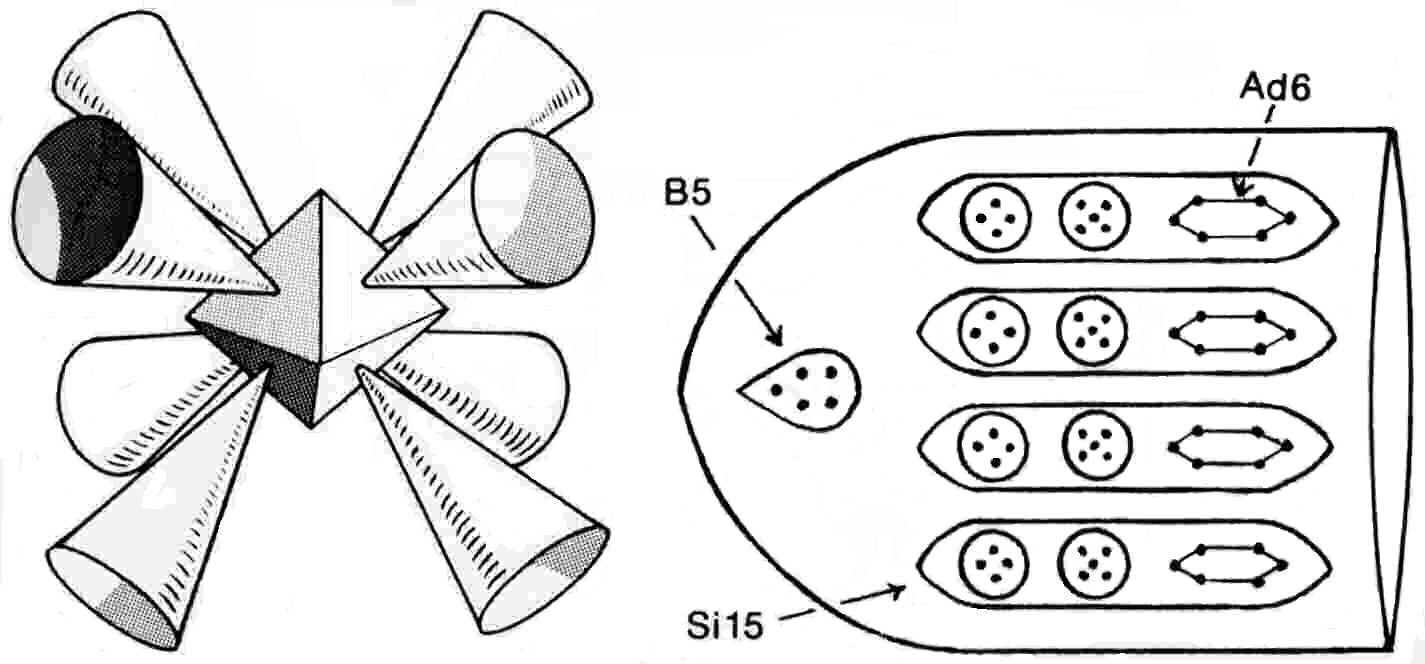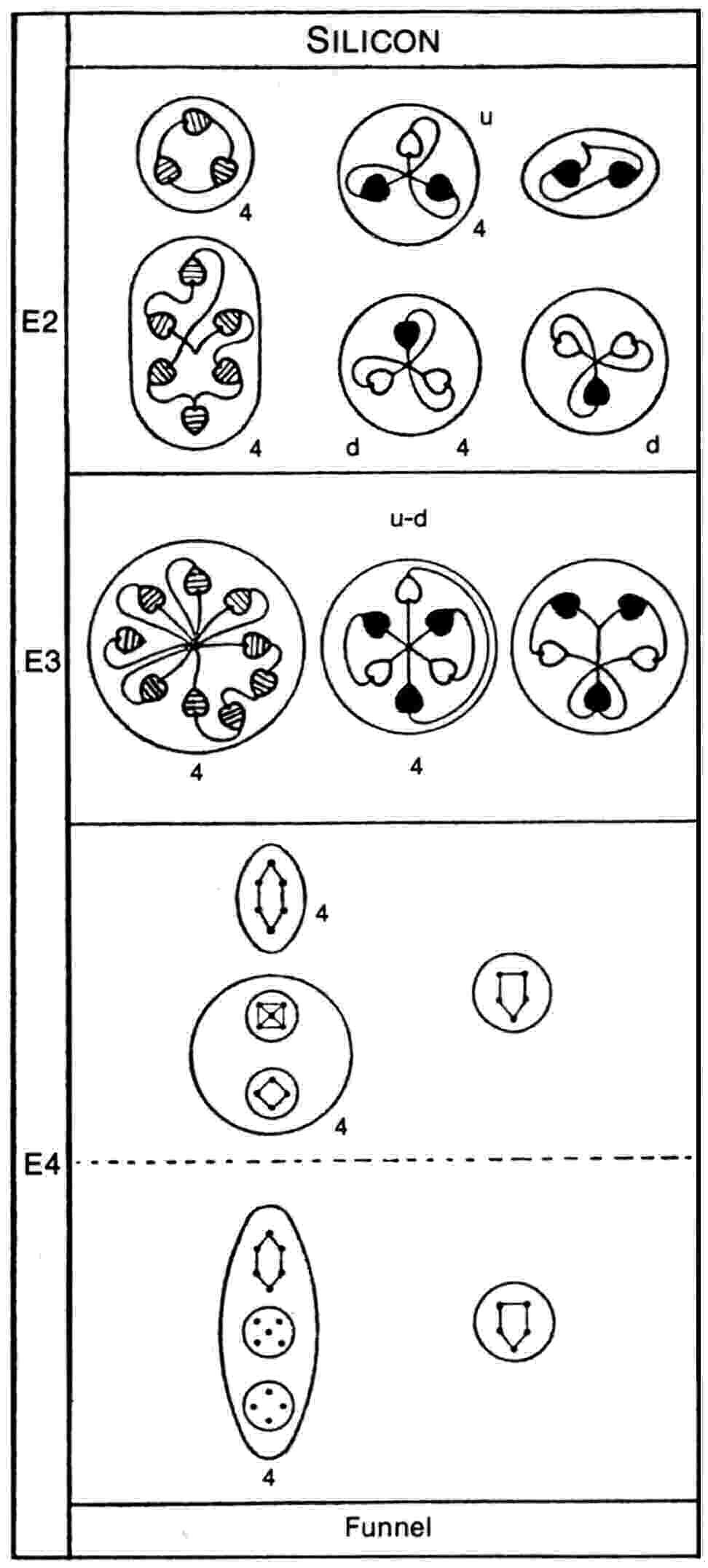The Silicon MPAThe silicon MPA consists of eight funnels directed towards the faces of an imaginary octahedron. Each funnel contains a B5 group and four ovoids (Si15), each enclosing an Ad6 group, a group of four UPAs and a group of five UPAs similar to that in the middle of an Al9′ group.

Silicon MPA = 8(B5 + 4Si15).

The silicon MPA is formed from two nuclei of Si28, the most abundant of the isotopes of silicon. They provide 504 subquarks — sixteen fewer than the number of UPAs. Thirty-two u quarks and thirty-two d quarks form thirty-two u-d diquarks (Ad6), four to a funnel.The disintegration diagram confirms that the four Ad6 groups are u-d diquarks because it shows that they break up at stage E2 into four (+) triplets (u quarks) and four (−) triplets (d quarks). The B5 groups in four funnels are bound states of three X subquarks and two Y subquarks, in agreement with the analysis of the boron MPA. The disintegration diagram confirms this by indicating that a B5 group breaks up at the E2 stage into a (−) triplet (d quark) and a (+) duad (X-X):

3X-2Y → d quark (= X-2Y) + X-X.

In the four other funnels, they are the mirror state B5′, which is a bound state of two X subquarks and three Y subquarks. The group of four UPAs is a 2X-2Y bound state. This predicted composition cannot be checked by means of the disintegration diagram because it indicates that the group of four UPAs combines with the group of five UPAs at the E3 stage, the resulting temporary state then breaking up into a group of six UPAs and a triplet of UPAs, so that the break-up of the group of four UPAs was never directly observed.

In two Si15 ovoids belonging to each of four funnels, the group of five UPAs consists of three X subquarks and two Y subquarks; in the other two ovoids, there should be two quartets of UPAs (either two 2X-2Y or one 4X and one 4Y). In the other four funnels, the group of five UPAs in two Si15 groups consists of three Y subquarks and two X subquarks, i.e., it is the mirror state of that in the other set of four funnels, whilst the two other Si15 ovoids also contain quartets of UPAs (either 2Y-2X or one 4Y and one 4X). The particles in four funnels are the mirror states of their counterparts in the four remaining funnels. Sixteen too many UPAs are present in the MPA due to an overcounting of two UPAs in each funnel, there being two groups of five UPAs and two groups of four UPAs instead of four groups of five UPAs in the Si15 ovoids of each funnel. Such an error could have occurred if Besant & Leadbeater, having examined one Si15 ovoid in detail, made only a cursory observation of the others, having assumed that they were similar, thereby overlooking the absence of one UPA in two of them.

Home